## Thompson抽样算法基本原理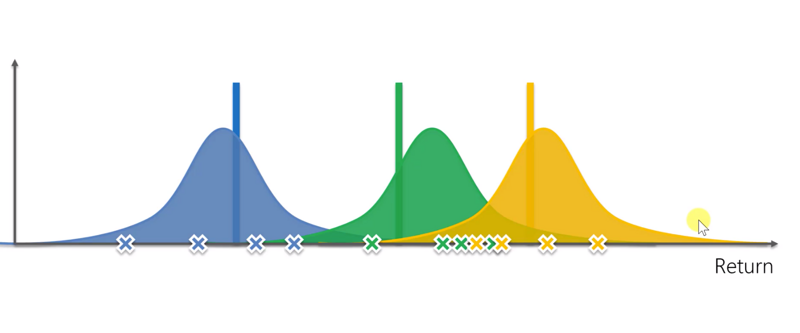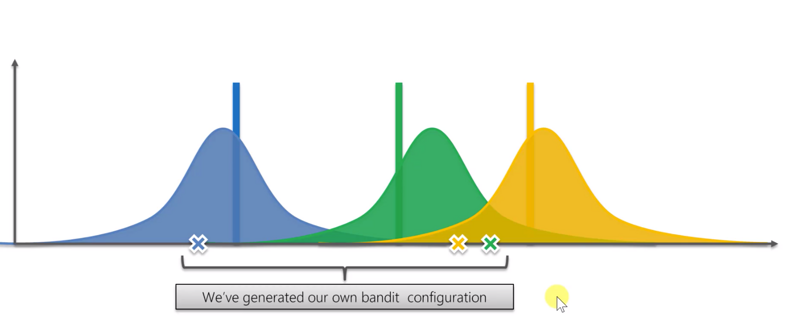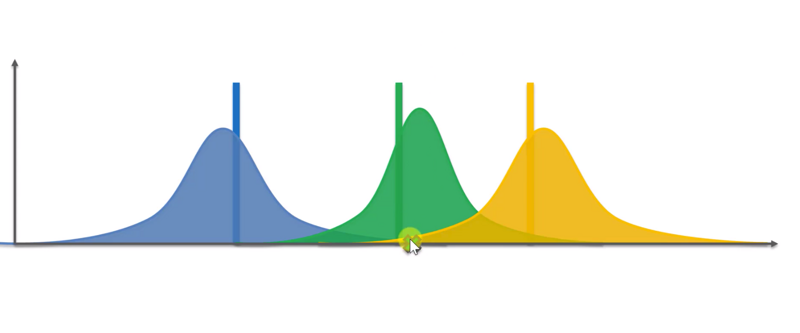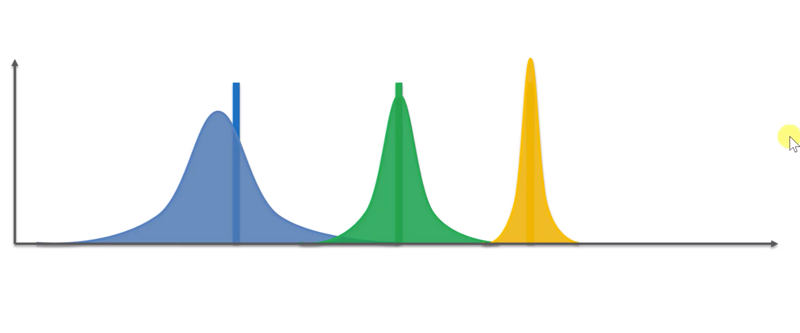## Thompson抽样算法 vs. 置信区间上界算法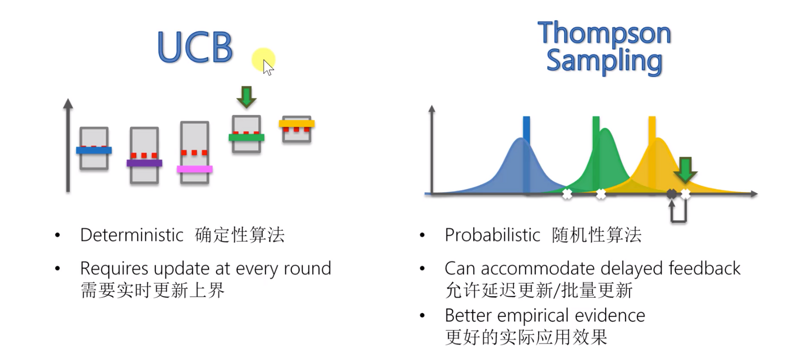## 代码实现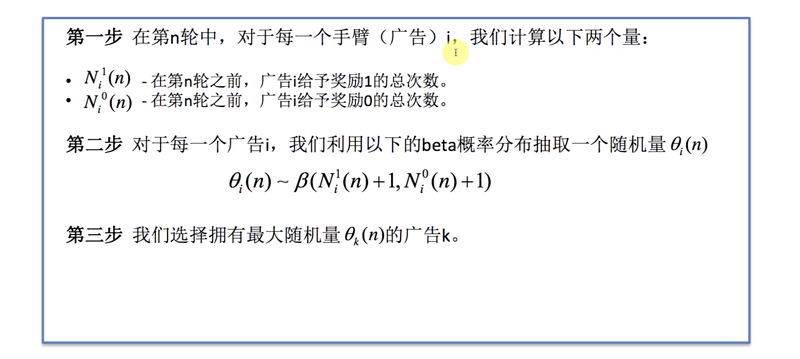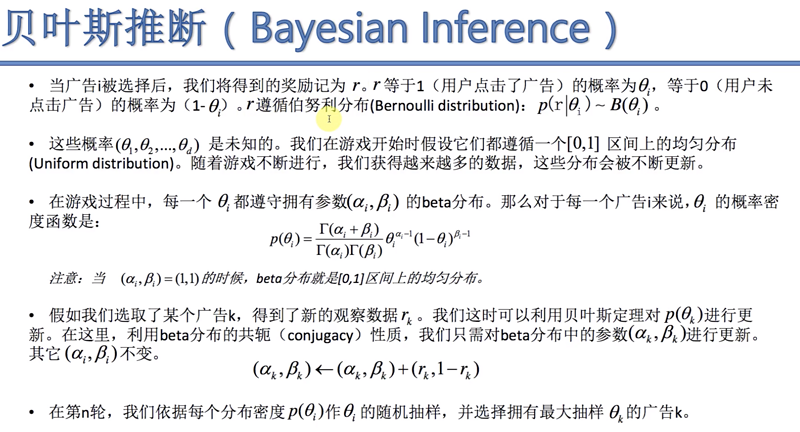import matplotlib.pyplot as plt
import pandas as pd
import random

# import the dataset

# Implementing Thompson Sampling
N = 10000
d = 10
numbers_of_rewards_1 =  * d
numbers_of_rewards_0 =  * d
total_reward = 0
for n in range(0, N):
max_random = 0
for i in range(0, d):
random_beta = random.betavariate(numbers_of_rewards_1[i] + 1, numbers_of_rewards_0[i] + 1)
if random_beta > max_random:
max_random = random_beta
if reward == 1:
else: